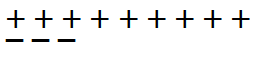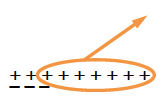Home > CC2MN > Chapter cc23 > Lesson cc23.2.1 > Problem3-34

3-34.The number $6$ is represented with $+$ and $-$ tiles at right. For each problem below, start with this diagram and then show how to remove the appropriate number of tiles to represent the problem given. State the final answer for each part. Homework Help ✎

1. $6-7$

The diagram has been drawn for you. Remember to state the final answer.1. $6-(-2)$

The answer is $8$. Remember to draw your diagram.

1. $6-3$

This one is very similar to part (a).